Popular tags: Support, Resistance, Trend, Range, Fibonacci, Wave, Divergence, Multi time frames(MTF), Swing, Volume, Overbought, Oversold

Featured

# Bullish XABCD 5-point M shape chart pattern indicator for Ninjatrader 8.

Availability: In Stock
Price: \$240.00USD \$219.00USD
Qty:     - OR -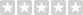(0/5)  0 reviews  |  Write a review

This is a life time license, no subscription or renew fee.

### Please make sure you understand the mechanism before making purchase.

XABCD 5-point chart patterns are very common and popular in technical analysis, some of them have already been named, such as Gartley, Butterfly, Bat, Crab, Shark and Cypher.

As the name suggests, XABCD pattern has 5 points and 4 legs formed by those 5 points. The concept of XABCD 5-point pattern is to compare the retracement level between legs. For example, when you see AB makes a 0.382 or 38.2% Fibonacci retracement of the X-A leg or XA, it means that the price difference from point A to B is equal to 38.2% of the price difference from point X to A.

If we use a formular to represent the relation, it will be: (B-A)=0.382*(X-A);

This indicator is able to detect and highlight any Bullish M shape XABCD 5-point chart pattern based on your pre-defined settings, and plots the fibonacci retracement automatically.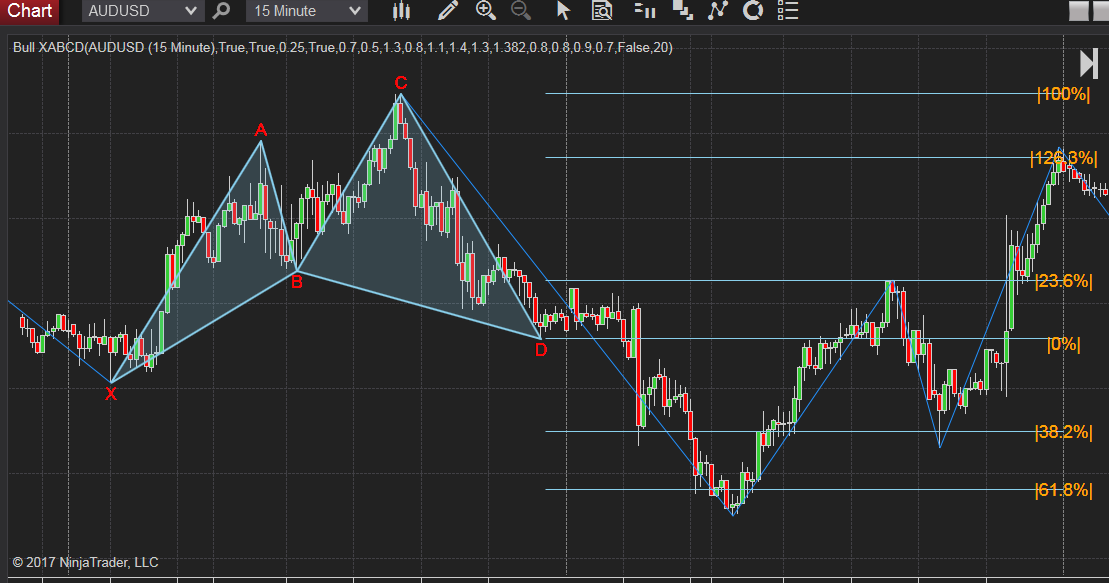The following video shows how it plots in realtime.

How it works:

It uses Zigzag to track the price trend and detect all four legs based on your settings. If there are four adjacent legs that meet the retracement levels you set, it will highlight the XABCD 5 points, plot two triangles and draw the Fibonacci retracemen lines. The image below is an example of what this indicator plots on chart.

There are two Zigzag deviation type:  percentage and point. The parameter "deviation value" is the actual number used for deviation type.
When you use percentage as the deviation type, it means that the value difference between two adjacent Zigzag point must be greater than the input percentage  which is the deviation value. For example, if you used percentage deviation type and set deviation value to 0.1, the value difference between X and A must greater than 0.1%. It also applies to all adjacent Zigzag points such as: A and B, B and C, C and D.

When you use point as the deviation type, it means that the value difference between two adjacent Zigzag point must be greater than the input point which is the deviation value. The value of Point is the actual price amount, if you set deviation value to 1 the value difference between X and A must greater than \$1. It also applies to all adjacent Zigzag points such as: A and B, B and C, C and D.
For example, if you used percentage deviation type and set deviation value to 0.1, the value difference between X and A must greater than \$0.1.

Please make sure you set the proper input for zigzag, otherwise you may not be able to detect the desired patterns.
You may need to use relatively small deviation value for Forex.Features:

1. Automatically highlight the M shape XABCD 5 points pattern and shade the triangle XAB and BCD.
2. You will be able to change the outline color for those 4 legs, XA, AB, BC and CD.
3. You will be able to change the shade color and transparent level of the two triangles XAB and BCD.
4. Automatically draw Fibonacci retracement levels with ON/OFF switch,
• adjust how many bars used for the extension of fibo levels.
• select Right or Left side to show the fibo values.
5. Plot a projection (not prediction) of the D point when XABC is confirmed.
• Enable to turn this feature ON/OFF.
• If the actual D point is detected, the projection will be removed.
6. Show XABCD patterns on history data, if turn this feature OFF, it will only plot the last one XABCD pattern.
7. Sound alert whenever a XABCD pattern is detected.
• Zigzag deviation type:  percentage or point
• Zigzag deviation value.
• Switch ON/OFF to show Zigzag line.The retracement levels in some of the XABCD patterns definition are theoretical values, it's not realistic to set the retracement as a fixed value such as 0.618, since you may not be able to find any pattern in a short time.

It's better to set the value as a range. For example, if you want to detect 0.618 retracement from AB to XA, then set a range from 0.6 to 0.64 will be more practical.

Set the inputs as:  ratio AB:XA <=0.6 and ratio AB:XA >=0.64.

Define the retracement between legs as shown in the picture below.

• If you want to compare BC and XA instead of BC and AB then enalbe BC:XA, it will disable BC:AB automatically. Same for CD:XC.Show XABCD patterns on history data, if turn this feature OFF, it will only plot the latest XABCD pattern.Otherwise, it will keep all qualified patterns on chart.

But only the last one will show Fibonacci retracement levels.The following chart is a pattern on history data.

It will not plot retracement levels on historical patterns.The following chart is an example of how projection mode works.
It will draw the piont D once point C is formed, the shade color is orange instead of lightblue.The following chart is an example of how customized XABCD ratio works.

You can set the ratios to any value you want.

I set the ratios of AB:XA and CD:BC to a range around 1, then the shape will be a M top or double top pattern as shown in below.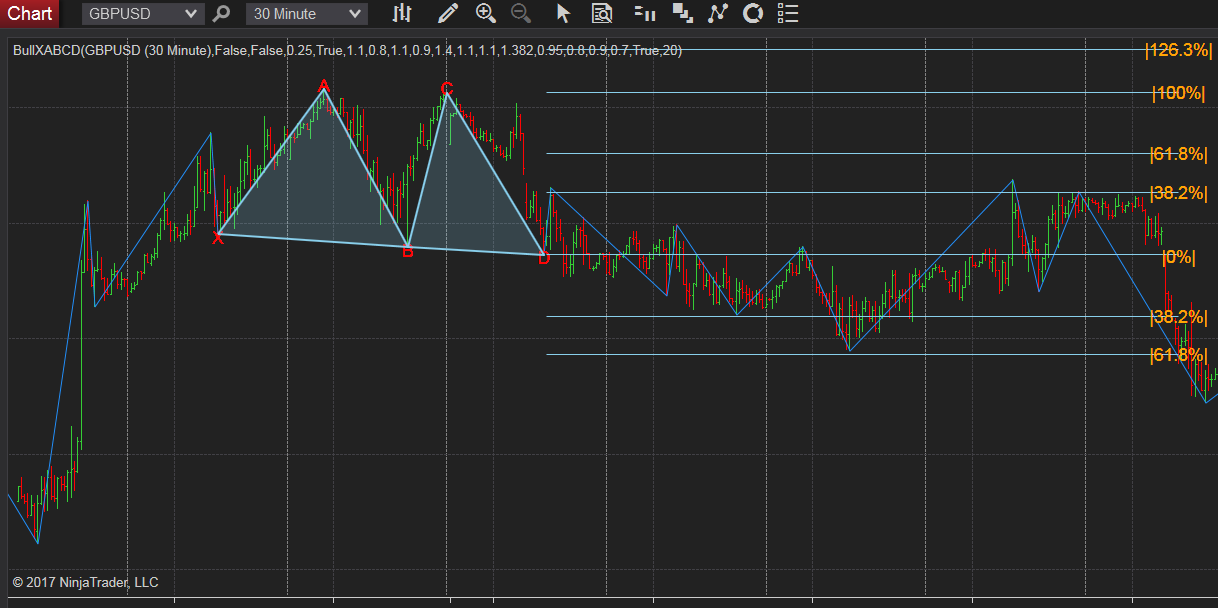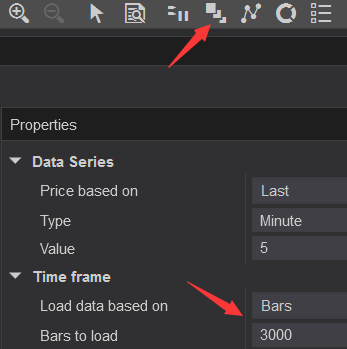You can also use this indicator to capture any XABCD pattern as well as the ones that already been named.

The following example shows how to use this indicator to detect Cypher Pattern.

Cypher Pattern Rules:

• The Cypher pattern starts with the X and A points.
• Point B is confirmed when AB retraces to 0.382 – 0.618 Fibonacci level of the leg XA
• Point C is confirmed when BC extend the XA leg by at least 1.272 or within 1.130 – 1.414 Fibonacci extension level
• Point D is confirmed when CD retraces 0.782 Fibonacci level of XC

The image below is the parameters for bullish Cypher Pattern, since it uses BC:XA and CD:XC, you need to enable those settings.The following example shows how to use this indicator to detect bullish Gartley pattern.

Gartley pattern rules:

1. Leg AB should be the .618 retracement of leg XA.
2. Point C is confirmed when BC retraces either .382 or .886 of AB.
3. If the retracement of BC is .382 of AB, then CD should be 1.272 of BC. If BC is .886 of AB, then CD should extend 1.618 of BC.
4. CD should be .786 retracement of XA.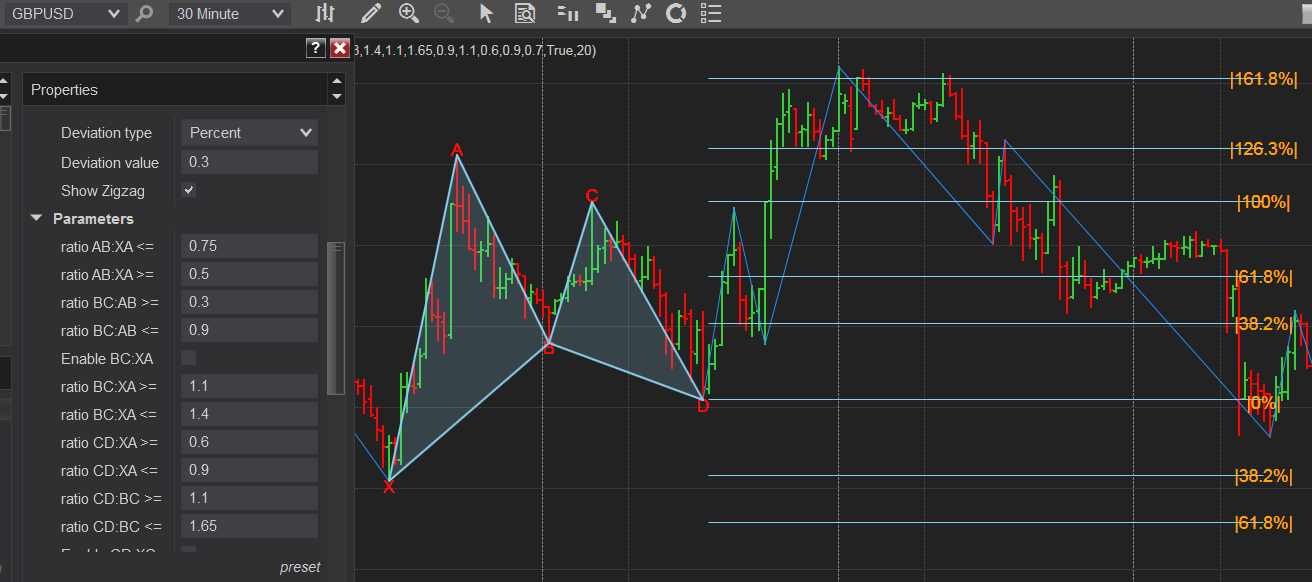The following example shows how to use this indicator to detect bullish Butterfly  pattern.

Butterfly pattern rules:

1. Move AB should be the .786 retracement of move XA.
2. Move BC can be either .382 or .886 retracement of move AB.
3. If the retracement of move BC is .382 of move AB, then CD should be 1.618 extension of move BC. Consquently, if move BC is .886 of move AB, then CD should extend 2.618 of move BC.
4. CD should be 1.27 or 1.618 extension of move XA.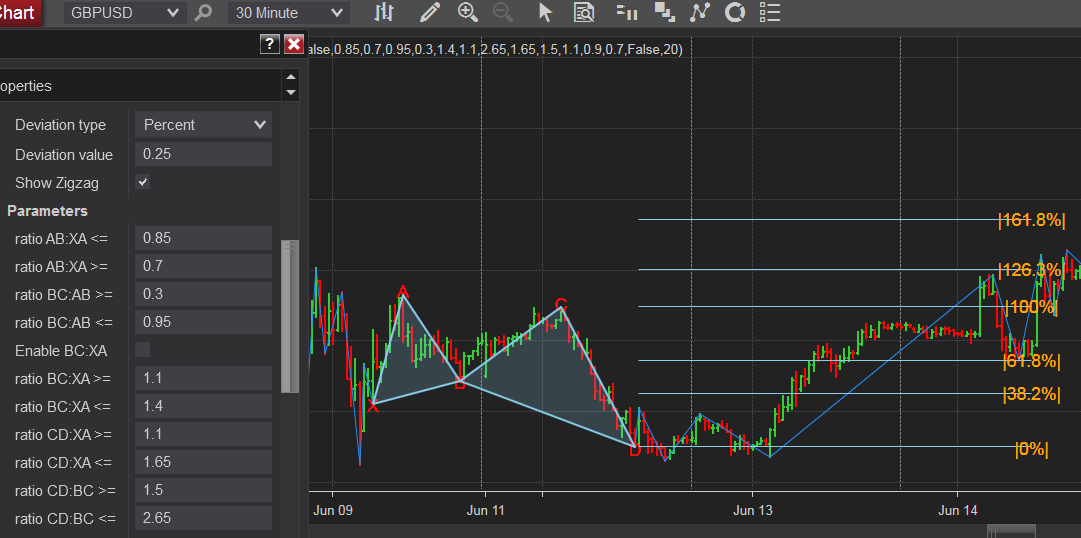Since the legs are based on Zigzag line, please make sure you set the proper input for zigzag first, otherwise you may not be able to detect the desired patterns.

This is a life time license, no subscription or renew fee.

## Write a review

Your Review: Note: HTML is not translated!

Enter the code in the box below:Tags: XABCD
Latest
Welcome to Patternsmart
Converting your indicator from one platform to another.